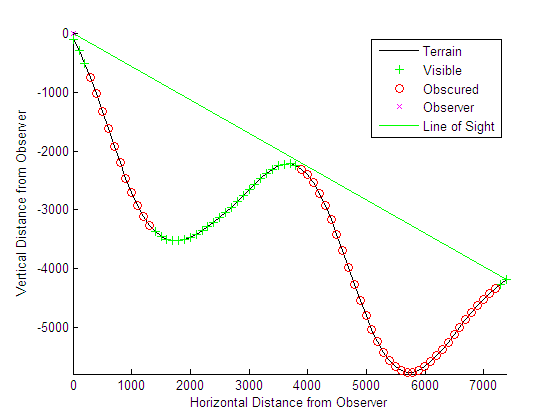## Determine and Visualize Visibility Across Terrain

You can use regular data grids of elevation data to answer questions about the mutual visibility of locations on a surface (intervisibility). For example,

• Is the line of sight from one point to another obscured by terrain?

• What area can be seen from a location?

• What area can see a given location?

The first question can be answered with the `los2` function. In its simplest form, `los2` determines the visibility between two points on the surface of a digital elevation map. You can also specify the altitudes of the observer and target points, as well as the datum with respect to which the altitudes are measured. For specialized applications, you can even control the actual and effective radius of the Earth. This allows you to assume, for example, that the Earth has a radius 1/3 larger than its actual value, a setting which is frequently used in modeling radio wave propagation.

### Compute Line of Sight

The following example shows a line-of-sight calculation between two points on a regular data grid generated by the `peaks` function. The calculation is performed by the `los2` function, which returns a logical result: `1` (points are mutually visible—intervisible), or `0` (points are not intervisible).

1. Create an elevation grid using `peaks` with a maximum elevation of 500, and set its origin at (0°N, 0°W), with a spacing of 1000 cells per degree):

```map = 500*peaks(100); maplegend = [ 1000 0 0];```
2. Define two locations on this grid to test intervisibility:

```lat1 = -0.027; lon1 = 0.05; lat2 = -0.093; lon2 = 0.042;```
3. Calculate intervisibility. The final argument specifies the altitude (in meters) above the surface of the first location (`lat1`, `lon1`) where the observer is located (the viewpoint):

```los2(map,maplegend,lat1,lon1,lat2,lon2,100) ans = 1```The `los2` function produces a profile diagram in a figure window showing visibility at each grid cell along the line of sight that can be used to interpret the Boolean result. In this example, the diagram shows that the line between the two locations just barely clears an intervening peak.

You can also compute the viewshed, a name derived from watershed, which indicates the elements of a terrain elevation grid that are visible from a particular location. The `viewshed` function checks for a line of sight between a fixed observer and each element in the grid. See the `viewshed` function reference page for an example.

## SupportGet trial now